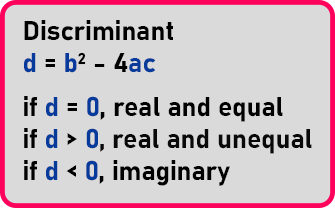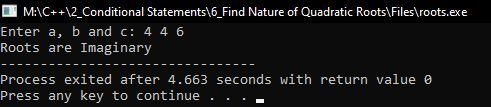# Find Nature of Quadratic Roots in C++

## Find Nature of Quadratic Roots in C++:

In this article, I am going to discuss How to Find the Nature of Quadratic Roots in C++ with Examples. Please read our previous article where we discussed Nested If Conditional Statement in C++ with Examples.

##### Find Nature of Quadratic Roots in C++

Here, we will check the nature of the roots of the quadratic equation first.Here we have a quadratic equation, it is an equation whose degree is two is called a quadratic equation. Below is the formula for the roots of the quadratic equationNow if you know the values of ‘a’, ‘b’, and ‘c’ then you can substitute them here and you can get the root. And for getting the root, first, we take ‘-b +’ and then we will take ‘-b –’. So, actually, we get two roots because this is quadratic.So, both the roots are the same, this means when the value of ‘b2 – 4ac’ is zero. Let’s say we have ‘d = -5’, so can you know the root of ‘-5? No, we cannot find the route of ‘-5’, because for negative ‘d’ the roots are imaginary. So, it means we cannot know the roots for negative ‘d’. We can call them imaginary roots.

1. If ‘d’ is zero, roots are the same only.
2. If ‘d’ is negative we cannot find the roots.
3. if ‘d’ is positive then we have two roots and they will be different.

This term ‘b2 – 4ac’ is known as Discriminant. So, ‘d’ is zero means roots are real and equal. if ‘d’ is greater than 0 means they are real but they are not unequal. If ‘d’ is less than 0 means they are not real but are imaginary.

So, if you have any quadratic equations, there are 3 different natures of the quadratic equation. There are 3 cases. So, we have to do nested if so let us write on the program directly.

##### Program for Nature of Quadratic Roots in C++:
```#include <iostream>
#include <math.h>
using namespace std;

int main()
{
float a, b, c, d, r1, r2;

cout << "Enter a, b and c: ";
cin >> a >> b >> c;

d = b * b - 4 * a * c;

if (d == 0)
{
cout << "Roots are real and equal";
cout << endl << (-b / (2 * a));
}
else if (d > 0)
{
cout << "Roots are real and unequal";
cout << endl << (-b + sqrt (d) / (2 * a));
cout << endl << (-b - sqrt (d) / (2 * a));
}
else
{
cout << "Roots are Imaginary";
}

return 0;
}
```
###### Output:In the next article, I am going to discuss How to Display Grades for Student Marks in C++ with Examples. Here, in this article, I try to explain How to Find the Nature of Quadratic Roots in C++ with Examples and I hope you enjoy this How to Find the Nature of Quadratic Roots in C++ with Examples article.